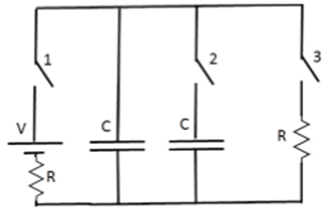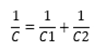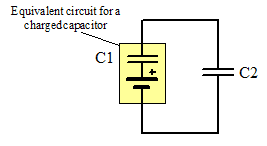# Electronic Circuits: Capacitors and switches

• Kosta1234
Actually they fit the definitions for both series and parallel connection; What is missing is some point of view or reference to decide whether to treat them as one or the other. All the circuit rules apply regardless.In summary, the voltage across the capacitor pairs is the same when they are connected in series, but not when they are connected in parallel.

## Homework StatementThey give the following circuit.
Each capacitor is 50*10^-6 [F], the Resistors are 2[Ω], and the Voltage is 5[V].

In the first step the close only the 1st switch and let the capacitor to be charged. I've found that the charge is 2.5*10^-6 [C]. (using the equation Q = V*C)
On the second part the OPEN the 1st switch and CLOSE the 2nd switch, so that the 3rd remains OPEN.
The question what is the charge on each of the capacitors on equilibrium?

Q = V*C

## The Attempt at a Solution

First of all, I don't understand what is happening with the Voltage source. Is he in the circuit? because the 1st switch is open
[/B]
Well, my attempt was that both of the Capacitors are now connected in series so that:so the total capacitance is (C/2)
so the total charge will be Q = V*(C/2), but it seems weird to me that the total charge will be less than the first capacitor in the beginning.

Thank you

#### Attachments

Your intuition is correct; the total charge after switch 1 is opened and switch 2 is closed should be the same as the total charge before. And since the two capacitors are identical, could one of them have more charge than the other?

I think it is not correct to say the capacitors are connected in series or in parallel since there is no battery in the circuit at that point.

•Delta2
Thank you!

I think it is not correct to say the capacitors are connected in series or in parallel since there is no battery in the circuit at that point.
Actually they fit the definitions for both series and parallel connection; What is missing is some point of view or reference to decide whether to treat them as one or the other. All the circuit rules apply regardless.

I will say, however, that you will find that treating them as parallel-connected will come in handy for these types of problems, particularly when the capacitors don't happen to have the same values and you need to find the voltage across the pair or the charge on each•Delta2
The capacitors will have the same current and the same voltage during the process that the left capacitor essentially charges the middle capacitor. So they seem to be both in series and parallel.

However when we say that the capacitors are in series we say that they will have the same charge (and not only the same current) at each instant of time of the process. But this does NOT hold here, the capacitors won't have the same charge at each instant of time( at start of time the left capacitor is fully charged and the middle has zero charge and gradually in time the charge of the left capacitor is decreased while the charge of the middle capacitor is increased). So they are not "exactly" in series. The equation 1/C=1/C1+1/C2 holds if they have the same charge during each instant of time but this is not true here so that equation cannot be used here.

To solve the problem just apply KVL at the closed loop AND conservation of charge. Do not bother with KCL, it is essentially useless in this problem.

Delta² said:
However when we say that the capacitors are in series we say that they will have the same charge (and not only the same current) at each instant of time of the process.
Not in general, no. That is only true (for capacitors) if they initially start with the same charge (often but not always it's zero).

The definitions of series and parallel connections are independent of the charge on the components: it's a circuit topology definition. The conclusions regarding current and voltage for series and parallel connected components are the result of applying Kirchhoff's laws to those topologies, and are not the starting point for the definitions. Those conclusions are, however, a very useful shortcut for analyzing many circuits.

gneill said:
Not in general, no. That is only true (for capacitors) if they initially start with the same charge (often but not always it's zero).

The definitions of series and parallel connections are independent of the charge on the components: it's a circuit topology definition. The conclusions regarding current and voltage for series and parallel connected components are the result of applying Kirchhoff's laws to those topologies, and are not the starting point for the definitions. Those conclusions are, however, a very useful shortcut for analyzing many circuits.
Ok fine, just that the equation 1/C=1/C1+1/C2 cannot be used here for the reason I said, the only reason that it gives the correct answer here is that the capacitors have the same capacitance (C1=C2 in this problem) so they will have the same charge at the end of the process. If we use different values for C1 and C2 and try to solve the problem using that equation we ll get wrong answer. The capacitors won't have the same charge during or at the end of process.

The charged capacitor can be replaced with an equivalent circuit comprised of an uncharged capacitor in series with a voltage source. The capacitance is the same value and the voltage source is equal to the potential across the original charged capacitor.You start knowing the initial voltage (and hence charge) on C1.

Then, taking the equivalent capacitance as 1/C=1/C1+1/C2, the ΔQ that occurs for each capacitor is the same and equal to

ΔQ = C⋅V

So ΔQ will be removed from the first capacitor and deposited on the second. You thus know the new charge distribution.

#### Attachments

gneill said:
The charged capacitor can be replaced with an equivalent circuit comprised of an uncharged capacitor in series with a voltage source. The capacitance is the same value and the voltage source is equal to the potential across the original charged capacitor.

View attachment 227239

You start knowing the initial voltage (and hence charge) on C1.

Then, taking the equivalent capacitance as 1/C=1/C1+1/C2, the ΔQ that occurs for each capacitor is the same and equal to

ΔQ = C⋅V

So ΔQ will be removed from the first capacitor and deposited on the second. You thus know the new charge distribution.
Nicely done I have to say but just to emphasize one thing:
$$|ΔQ_1| =| ΔQ_2| = C⋅V$$ that is correct but though the $$|ΔQi| = C⋅V$$ is the same for each capacitor , the final charge that each capacitor will have, will not necessiraly be the same because the initial charges are different and $$Q_i^{final}=Q_i^{initial}+ ΔQ_i$$. It is just a "conspiracy" that it happens to be the same here , and that is because C1=C2.

Last edited:
Delta² said:
It is just a "conspiracy" that it happens to be the same here , and that is because C1=C2.
Nope. It'll work with any values of capacitors. You just have to remember that the original charged capacitor had an initial charge that is "debited" by ΔQ. As I stated, you know the initial charge on C1, so you know the final charge on it when you subtract ΔQ.

gneill said:
Nope. It'll work with any values of capacitors. You just have to remember that the original charged capacitor had an initial charge that is "debited" by ΔQ. As I stated, you know the initial charge on C1, so you know the final charge on it when you subtract ΔQ.
yes that's the "trap" of this exercise, that the initial charges on each capacitor are not the same.

Delta² said:
yes that's the "trap" of this exercise, that the initial charges on each capacitor are not the same.
True enough. But in the end you can employ either the "parallel" or "series" paradigm to arrive at the same end result. It's perhaps a bit more tricky to use the series scenario since you need to have the insight to employ the equivalent circuit for a charged capacitor. This is not necessarily something expected of entry level students who are just getting familiar with circuit theory. Personally, I'd consider awarding bonus points if they went in this direction.

The parallel connection paradigm is the most often chosen for the path to solution for these sorts of problems. It ends up involving solving a pair of equations with two unknowns (the charges on the capacitors). I think that depending upon one's mathematical proclivities, one could argue either way as to which approach is more efficient.

•Delta2